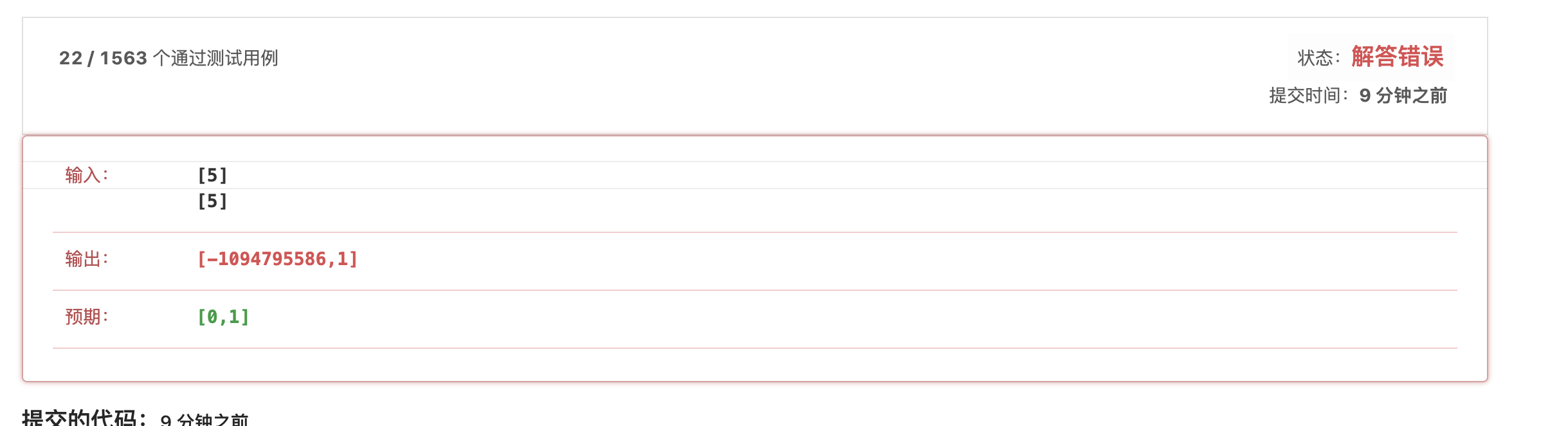struct ListNode* addTwoNumbers(struct ListNode* l1, struct ListNode* l2)
{
struct ListNode* p1 = l1;
struct ListNode* p2 = l2;
int val1 = 0, val2 = 0, count = 0, power = 0;

while(p1 != NULL)
{
val1 = val1 + pow(10,power) * p1->val;
power ++;
p1 = p1->next;
}
power = 0;
while(p2 != NULL)
{
val2 = val2 + pow(10,power) * p2->val;
power ++;
p2 = p2->next;
}
int total = val1 + val2;
int val3 = total;

struct ListNode* p = NULL;
struct ListNode* current = NULL;
struct ListNode* head = (struct ListNode*)malloc(sizeof(struct ListNode));
if(val3 == 0)
{
p = (struct ListNode*)malloc(sizeof(struct ListNode));
p->val = 0;
p->next = NULL;
}
else
{
while(total != 0)
{
total /= 10;
count ++;
}

int *v_arr = (int *)malloc(count*sizeof(int));
int i = 0, temp = count;
while(val3 != 0)
{
v_arr[i] = val3 / pow(10,temp-1);
val3 = val3 % (int)pow(10,temp-1);
i++;
temp--;
}

while(count != 0)
{
p = (struct ListNode*)malloc(sizeof(struct ListNode));
p->val = v_arr[count-1];
p->next = NULL;
current = p;
}
else{
current -> next = p;
current = p;
}
count --;
}
}